Intro to Addition Math Videos for Kids - Grades K-2
1%
It was processed successfully!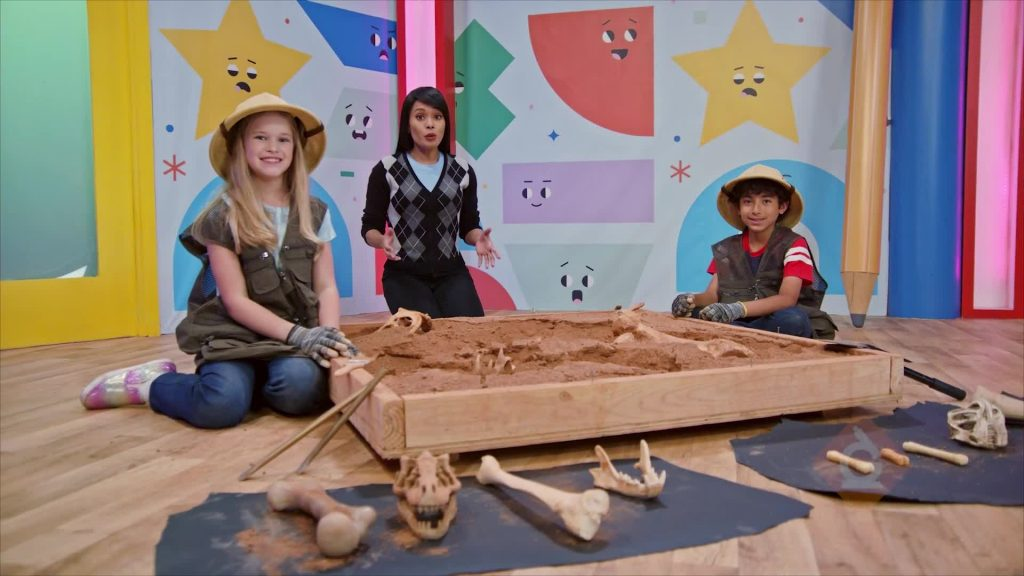Addition is when you put two groups together. When you add, you find the new total.

WHAT IS ADDITION?. Addition is when you put two groups together. When you add, you find the new total. To better understand addition…

## LET’S BREAK IT DOWN!

### You can count to find how many in all.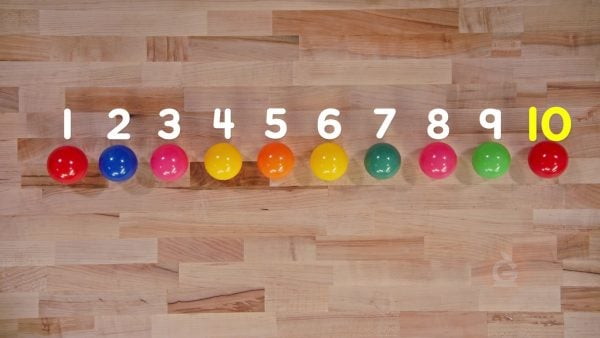You have a pile of balls. You can count the balls in the pile: 1, 2, 3, 4, 5, 6, 7, 8, 9, 10. There are 10 balls.

You can count to find how many in all. You have a pile of balls. You can count the balls in the pile: 1, 2, 3, 4, 5, 6, 7, 8, 9, 10. There are 10 balls.

### Count all of the kittens.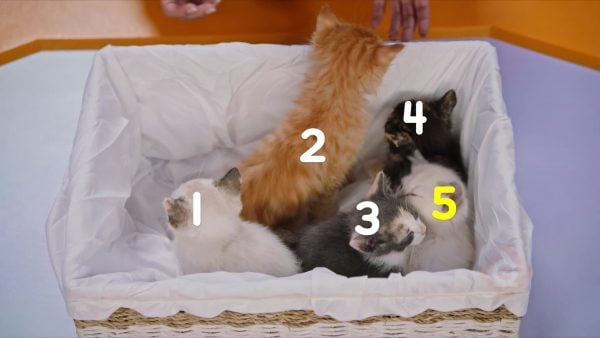There are 4 kittens. 1 more kitten joins them. How can you find how many kittens in all? Count all of the kittens: 1, 2, 3, 4, 5. There are 5 kittens in all.

Count all of the kittens. There are 4 kittens. 1 more kitten joins them. How can you find how many kittens in all? Count all of the kittens: 1, 2, 3, 4, 5. There are 5 kittens in all.

### Use + and = to show how many kittens.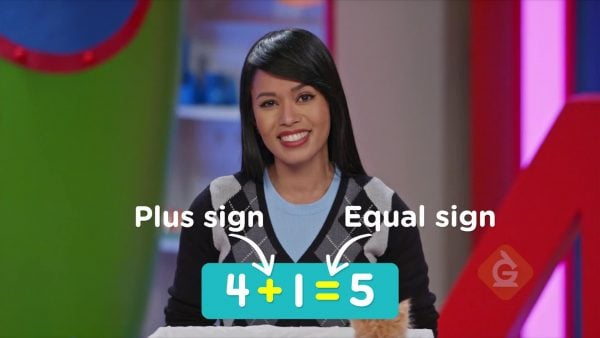A “+” shows two groups joined together. 4 + 1 = 5. The “+” shows that 4 kittens and 1 kitten are together. The “=” shows that 4 and 1 are equal to 5. There are 5 kittens in all.

Use + and = to show how many kittens. A “+” shows two groups joined together. 4 + 1 = 5. The “+” shows that 4 kittens and 1 kitten are together. The “=” shows that 4 and 1 are equal to 5. There are 5 kittens in all.

### Count on to count cupcakes.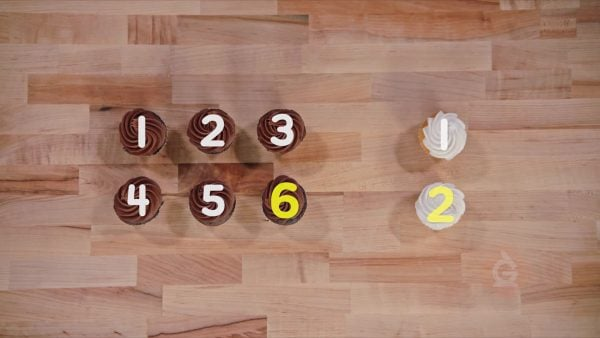There are 2 vanilla cupcakes and 6 chocolate cupcakes. Count on to find how many in all. Start at 6. Count up 2: 7, 8. You can also start at 2 and count up: 3, 4, 5, 6, 7, 8. There are 8 cupcakes in all. 2 + 6 = 8.

Count on to count cupcakes. There are 2 vanilla cupcakes and 6 chocolate cupcakes. Count on to find how many in all. Start at 6. Count up 2: 7, 8. You can also start at 2 and count up: 3, 4, 5, 6, 7, 8. There are 8 cupcakes in all. 2 + 6 = 8.

### Counting to find how many dinosaur bones.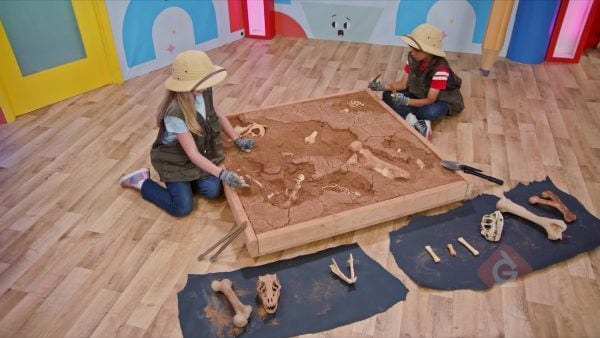You found 6 bones then 4 bones. How many bones did you find in all? You can count all of the bones: 1, 2, 3, 4, 5, 6, 7, 8, 9, 10. You can count on from 6: 7, 8, 9, 10. There are 10 bones in all. 6 + 4 = 10.

Counting to find how many dinosaur bones. You found 6 bones then 4 bones. How many bones did you find in all? You can count all of the bones: 1, 2, 3, 4, 5, 6, 7, 8, 9, 10. You can count on from 6: 7, 8, 9, 10. There are 10 bones in all. 6 + 4 = 10.

When one group joins another group to find how many you have all together.
Counting All
Counting how many in all starting with 1 to the total.
Plus Sign
The symbol that means addition, or joining two groups together.
Equals Sign
The symbol that means both sides have the same value.
Counting On
Counting how many in all when adding, starting at one of the numbers being joined, and counting the rest.
Sum

1, 2, 3, 4, 5

3, 4, 5

7

8

3 + 7 = 10
X

## Success

We’ve sent you an email with instructions how to reset your password.
Ok